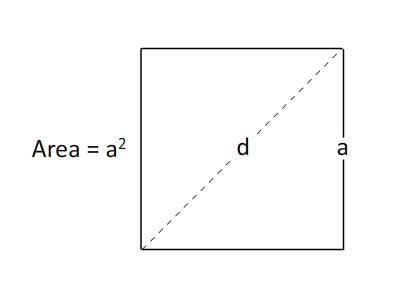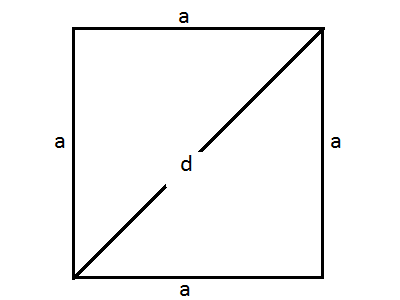# Calculate Square Area, Perimeter and Length of Diagonal

Square area calculator tool is used to calculate area, perimeter and length of the diagonal of a square. Enter length of the one side of a square to calculate all parameters.### FormulasThe formula for Area of a Square is: a2, where 'a' is the side of a square.

The formula for Perimeter of a Square is: 4 a

The formula for Length of the Diagonal of a Square (d) is: √2 a

#### What is a Square?

Square is a plane figure with four equal straight sides and four right angles.

#### Perimeter of a Square

A square is a kind of rectangle, and the perimeter of any rectangle is the sum of its four sides. Since all sides of a square are the same, perimeter=4a

#### Area of a Square

The area of a square is equal to any one of its sides times any other: a * a . Since that's the same as s squared, area=a2

#### Length of the Diagonal of Square

To find the length of the diagonal of a square, multiply the length of one side by the square root of 2.

### Facts About Square

• It is a polygon with 4 sides of equal length and 4 right angle corners (90 degree corners).
• Since, it has 4 sides of equal length, a square is a regular quadrilateral.
• It is also a rectangle with equal sides and a rhombus with right angles.
• The area of a square is equal to the length of one side to the power of two (length squared).
• Its perimeter is 4 times the length of one side.
• A square has a larger area than all other quadrilaterals with the same perimeter.
• The diagonals of a square bisect each other at 90 degrees and are perpendicular.
• Opposite sides are parallel.
• The internal angles add to 360 degrees.
• A square has 4 lines of reflectional symmetry.
• Square shapes are often used by humans for design and engineering purposes such as town planning.